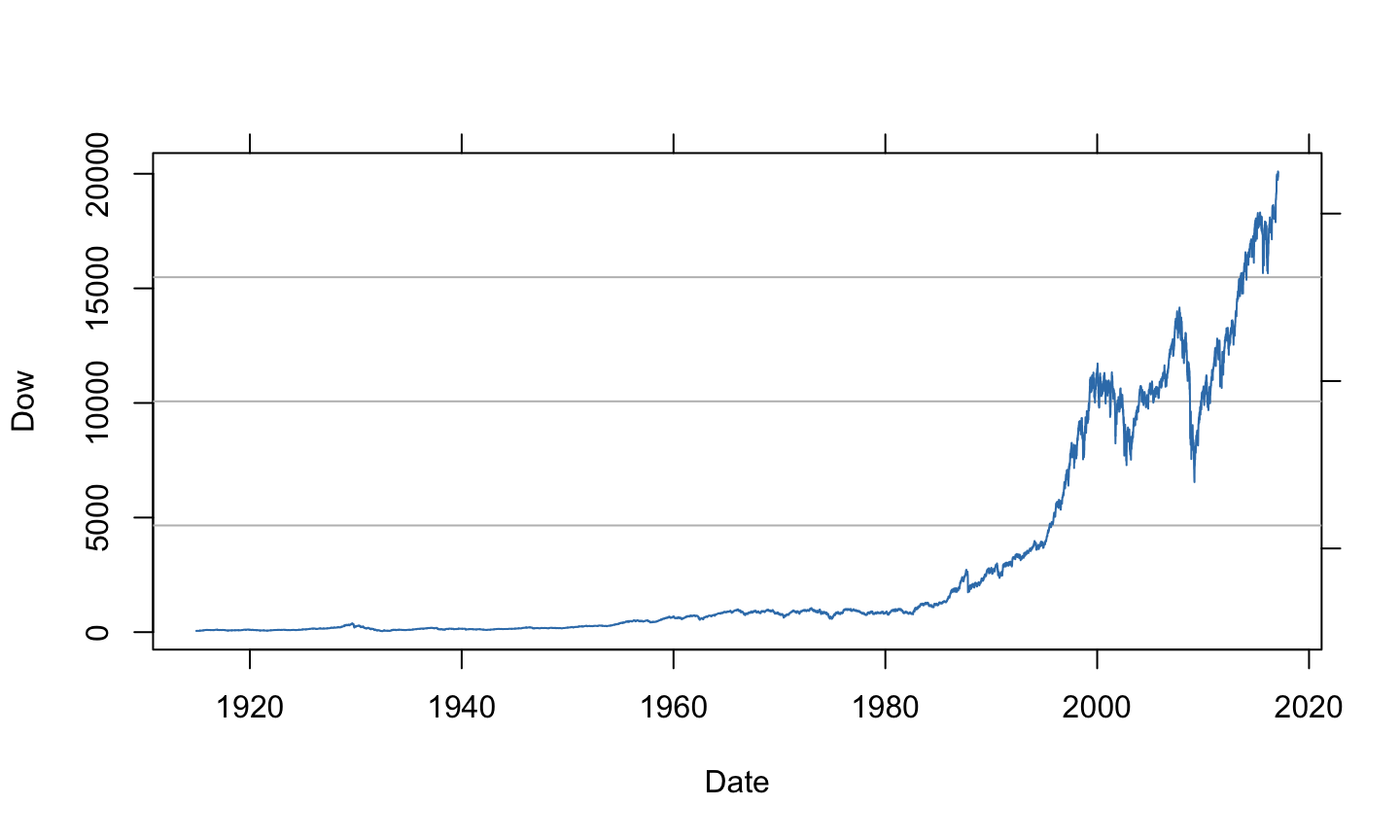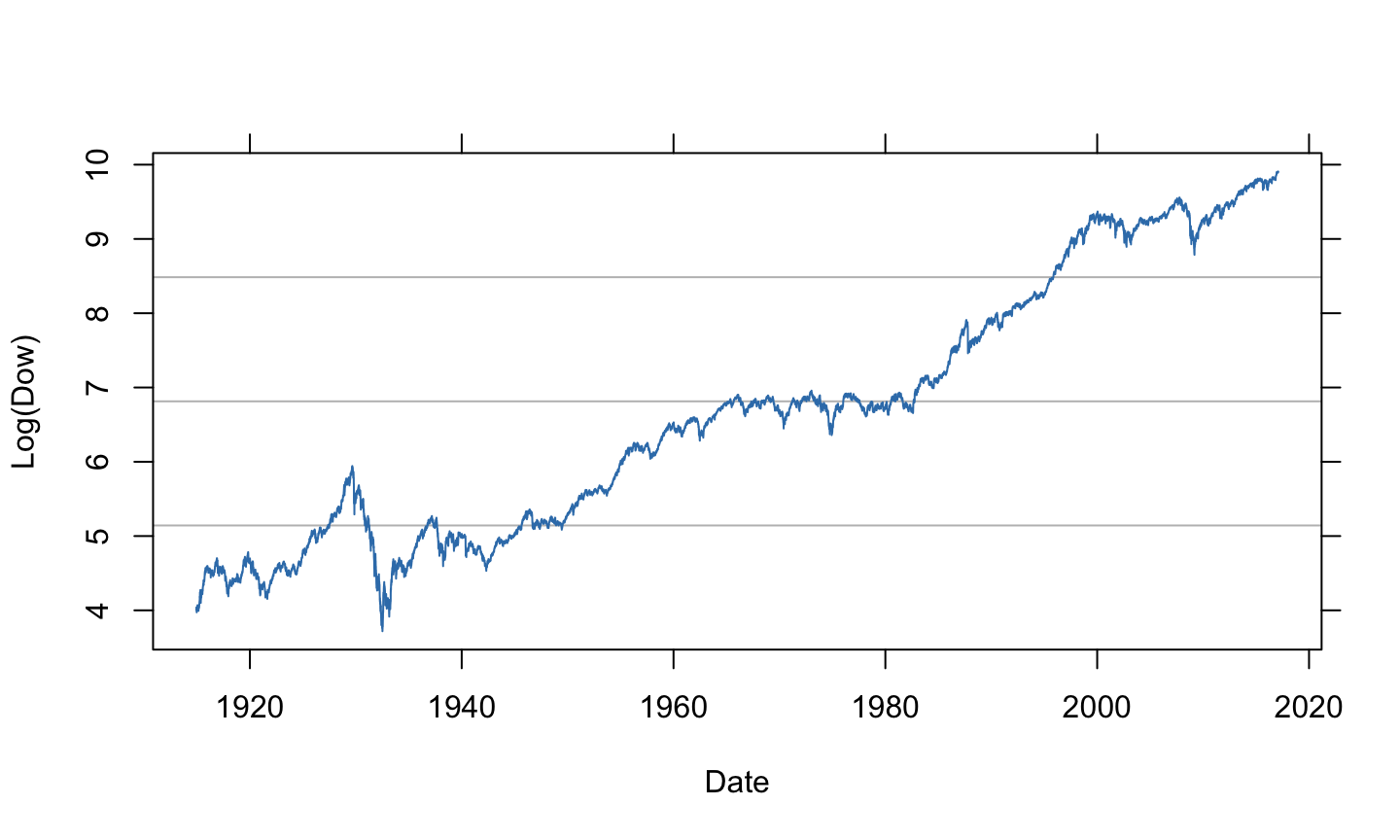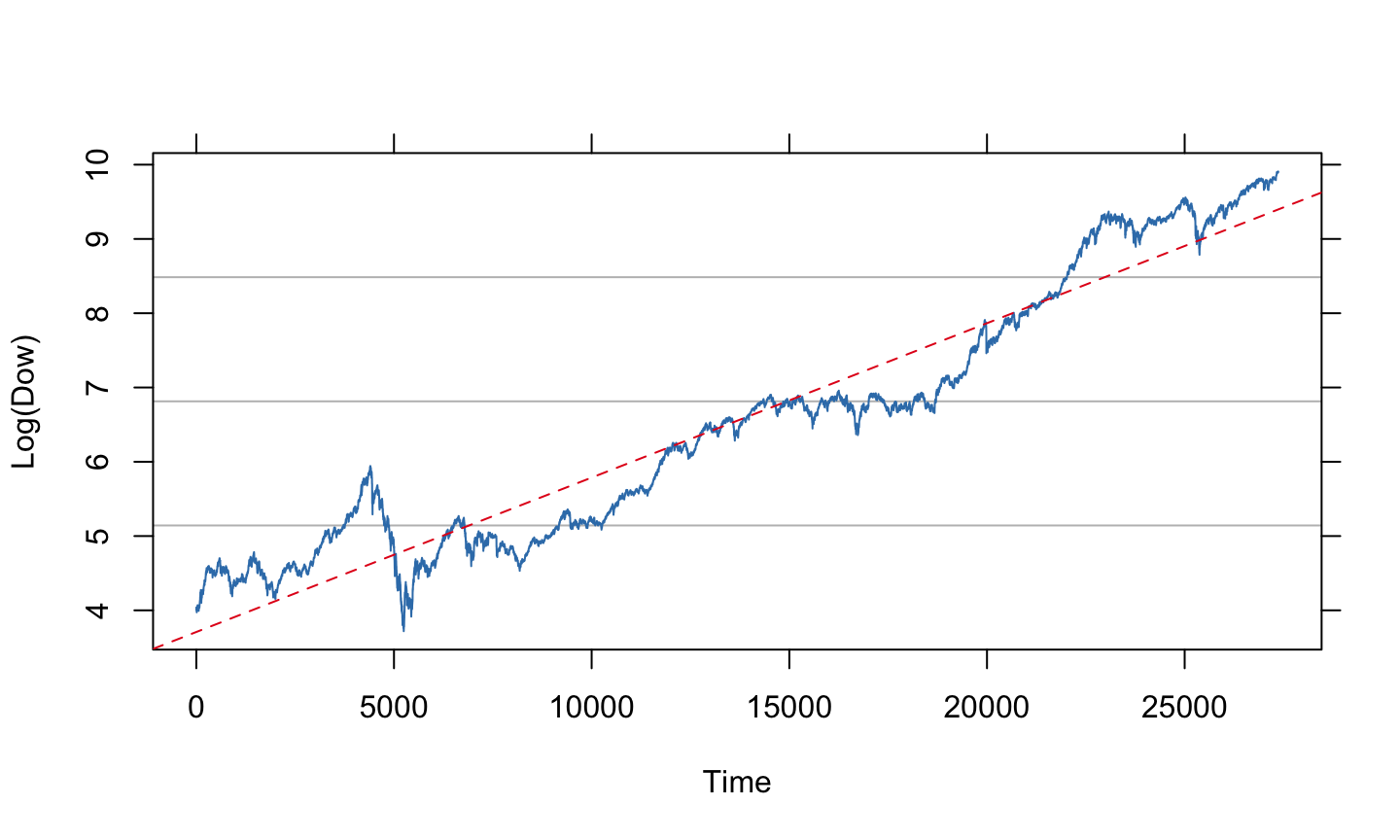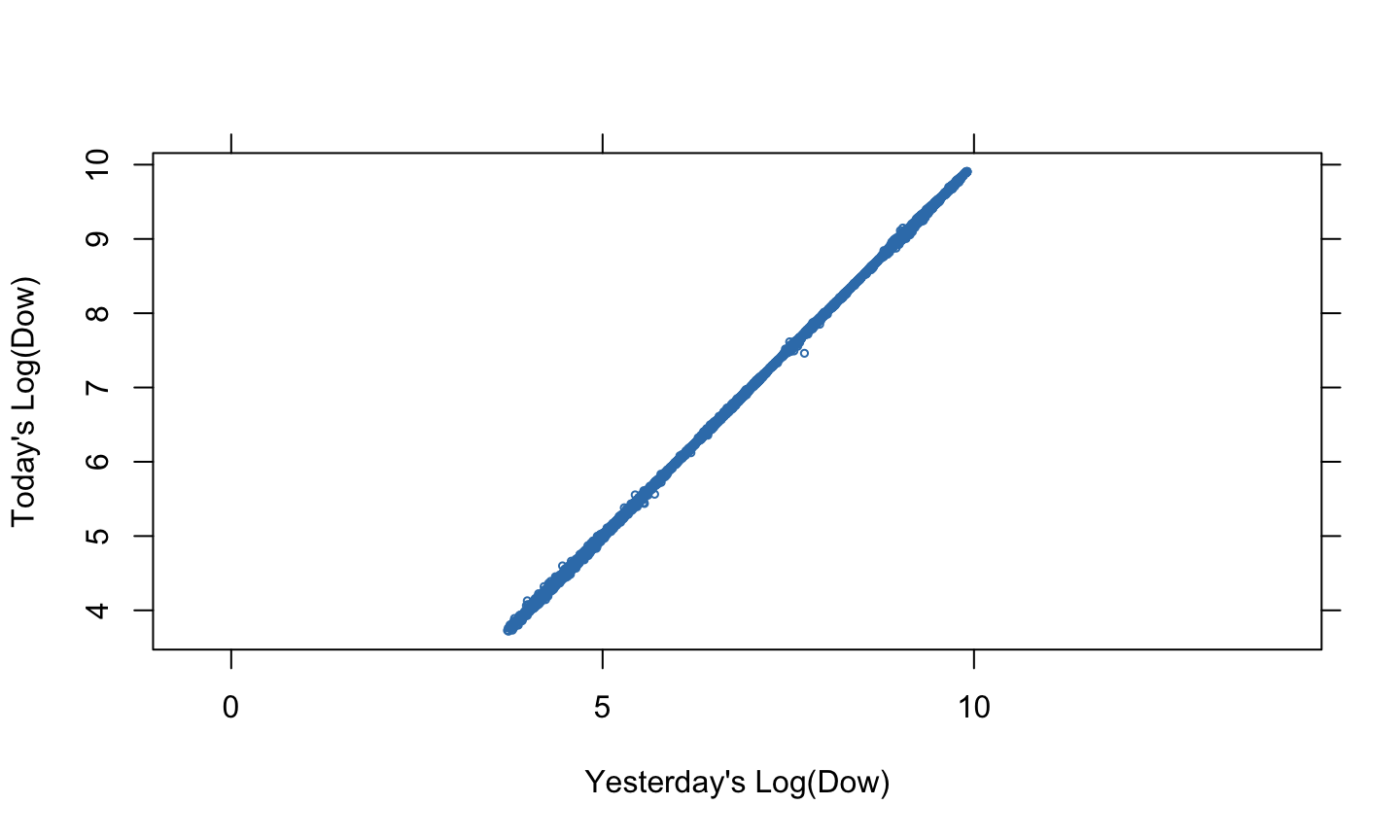February 7, 2017

## Dow Jones Industrial Average## Log(Dow)Take logs to adjust for exponential growth and level-dependent volatility.

## Stylized Fact #1

Many datasets exhibit exponential growth and level-dependent volatility.

## Regress Log(Dow) on Time

```Call:
lm(formula = log_dow ~ time)

Residuals:
Min       1Q   Median       3Q      Max
-1.08022 -0.40173 -0.02597  0.42103  1.31975

Coefficients:
Estimate Std. Error t value Pr(>|t|)
(Intercept) 3.708e+00  5.667e-03   654.5   <2e-16 ***
time        2.079e-04  3.586e-07   579.6   <2e-16 ***
---
Signif. codes:  0 '***' 0.001 '**' 0.01 '*' 0.05 '.' 0.1 ' ' 1

Residual standard error: 0.4687 on 27367 degrees of freedom
Multiple R-squared:  0.9247,    Adjusted R-squared:  0.9247
F-statistic: 3.36e+05 on 1 and 27367 DF,  p-value: < 2.2e-16```

## Log(Dow) vs. Time, with Fit## Today's vs. Yesterday's Log(Dow)## Regress Today on Yesterday

```Call:
lm(formula = log_dow ~ lag1_log_dow)

Residuals:
Min          1Q      Median          3Q         Max
-0.25654574 -0.00470490  0.00025780  0.00508635  0.14253642

Coefficients:
Estimate  Std. Error     t value Pr(>|t|)
(Intercept)  1.57303e-04 2.63292e-04     0.59745  0.55021
lag1_log_dow 1.00001e+00 3.88805e-05 25720.06238  < 2e-16 ***
---
Signif. codes:  0 '***' 0.001 '**' 0.01 '*' 0.05 '.' 0.1 ' ' 1

Residual standard error: 0.0109845 on 27366 degrees of freedom
(1 observation deleted due to missingness)
Multiple R-squared:  0.999959,  Adjusted R-squared:  0.999959
F-statistic: 6.61522e+08 on 1 and 27366 DF,  p-value: < 2.22e-16```

## Slope significantly different from 1?

```                 Estimate   Std. Error      t value  Pr(>|t|)
(Intercept)  0.0001573034 0.0002632925 5.974476e-01 0.5502135
lag1_log_dow 1.0000089250 0.0000388805 2.572006e+04 0.0000000```

T statistic:

` 0.2295497`

95% Confidence Interval:

```                2.5 %  97.5 %
lag1_log_dow 0.999933 1.00009```

We probably shouldn't trust these results. (Why?)

## Stylized Fact #2

Nearby observations are highly correlated with each other.

## Log(Dow) vs. Time (again)